# NCERT Exemplar Solutions for Class 6 Maths Chapter 3 Integers

NCERT Exemplar Solutions for Class 6 Maths Chapter 3 Integers covers all the exercise questions in the NCERT Exemplar textbook. We, at BYJU’S, ensure that you get the best NCERT Exemplar Solutions study material that can help you excel in your studies with a well-organised way of learning. These NCERT Exemplar Solutions for Class 6 Maths are prepared by our well-trained and qualified subject experts and are provided here to assist students in learning the concepts quickly and with precision.

Chapter 3 – Integers is a whole number that can be positive, negative, or zero. This chapter covers topics like the addition of two negative integers, the addition of two positive integers, additive inverse, comparing two integers on the number line. Students can also see how one more than a given number gives a successor and one less than the given number gives a predecessor.

## Download the PDF of NCERT Exemplar Solutions for Class 6 Maths Chapter 3 Integers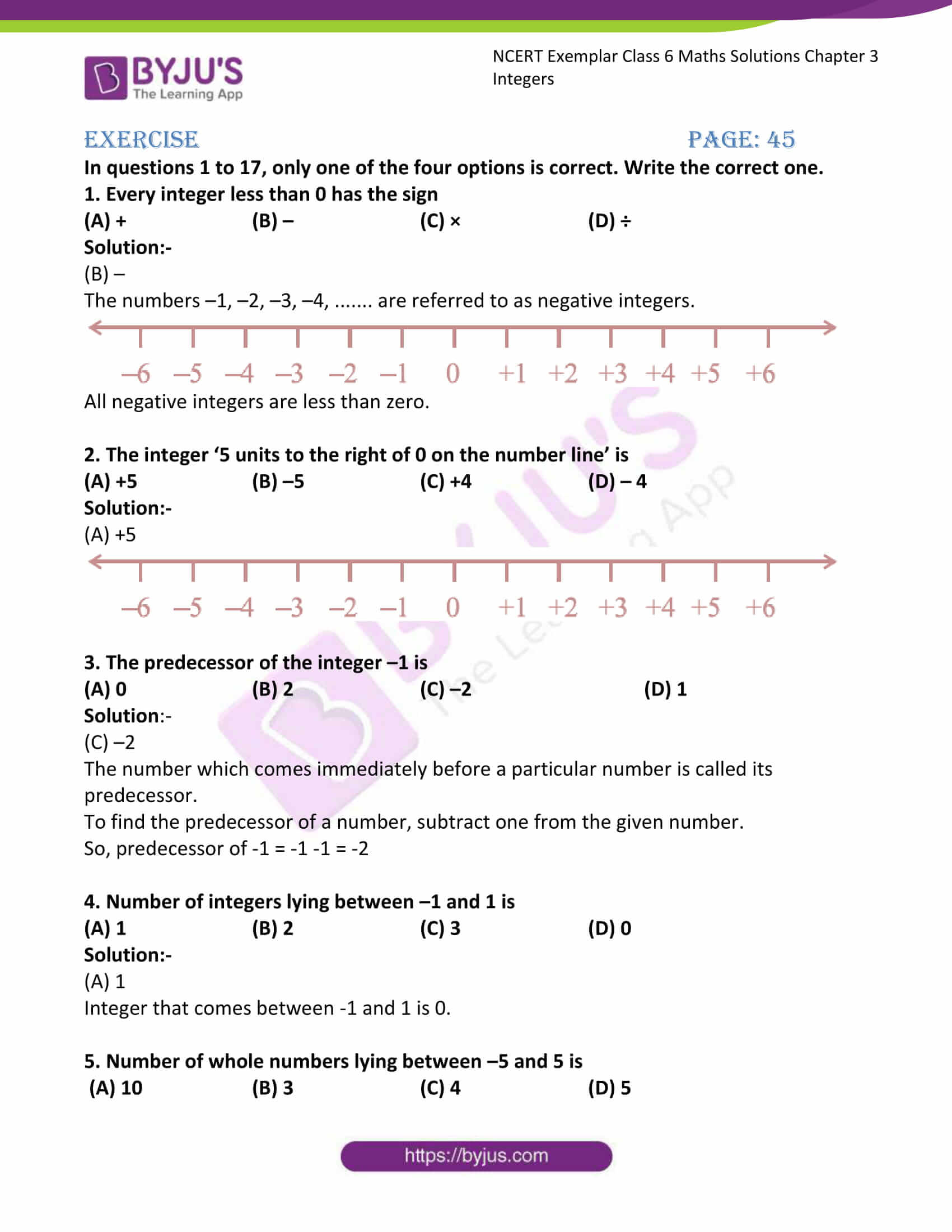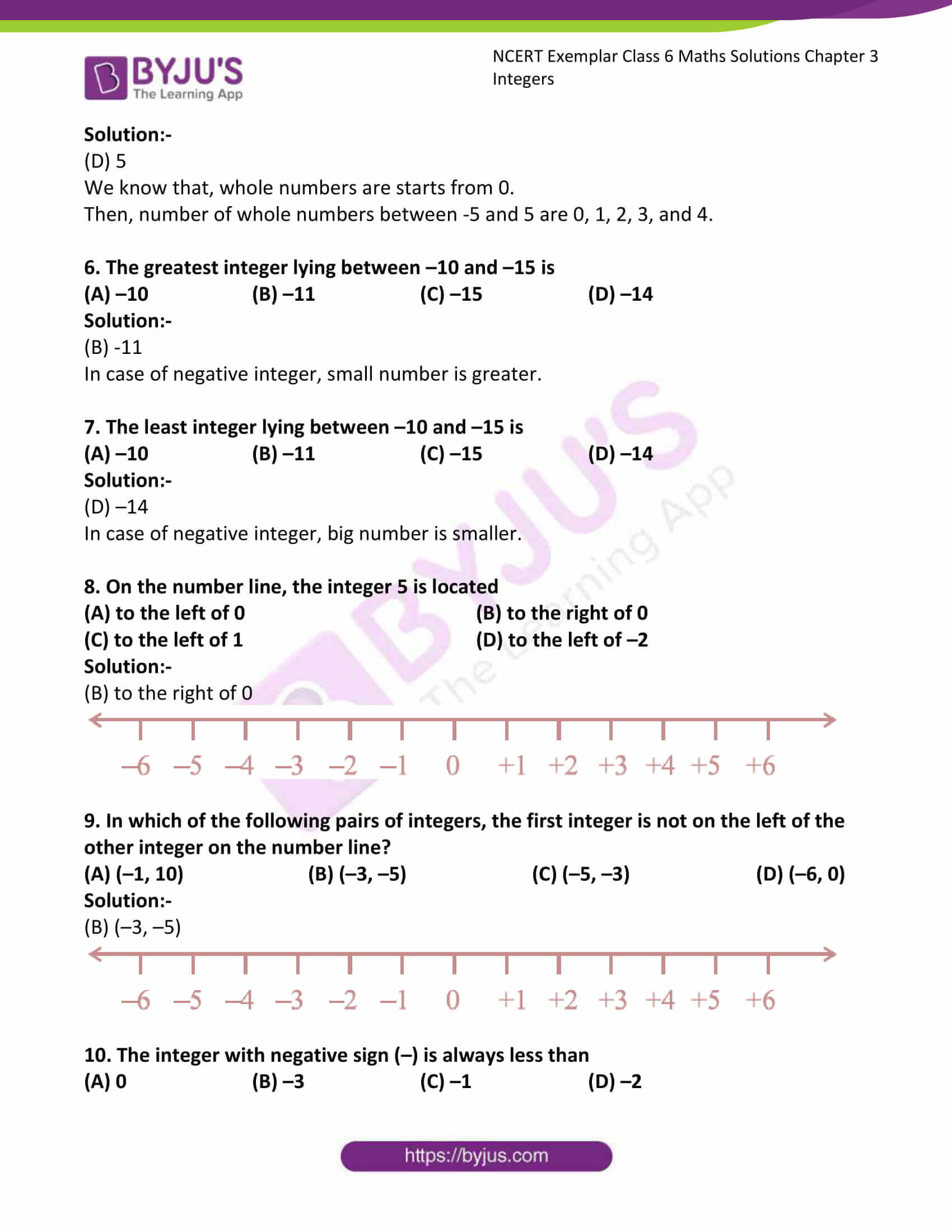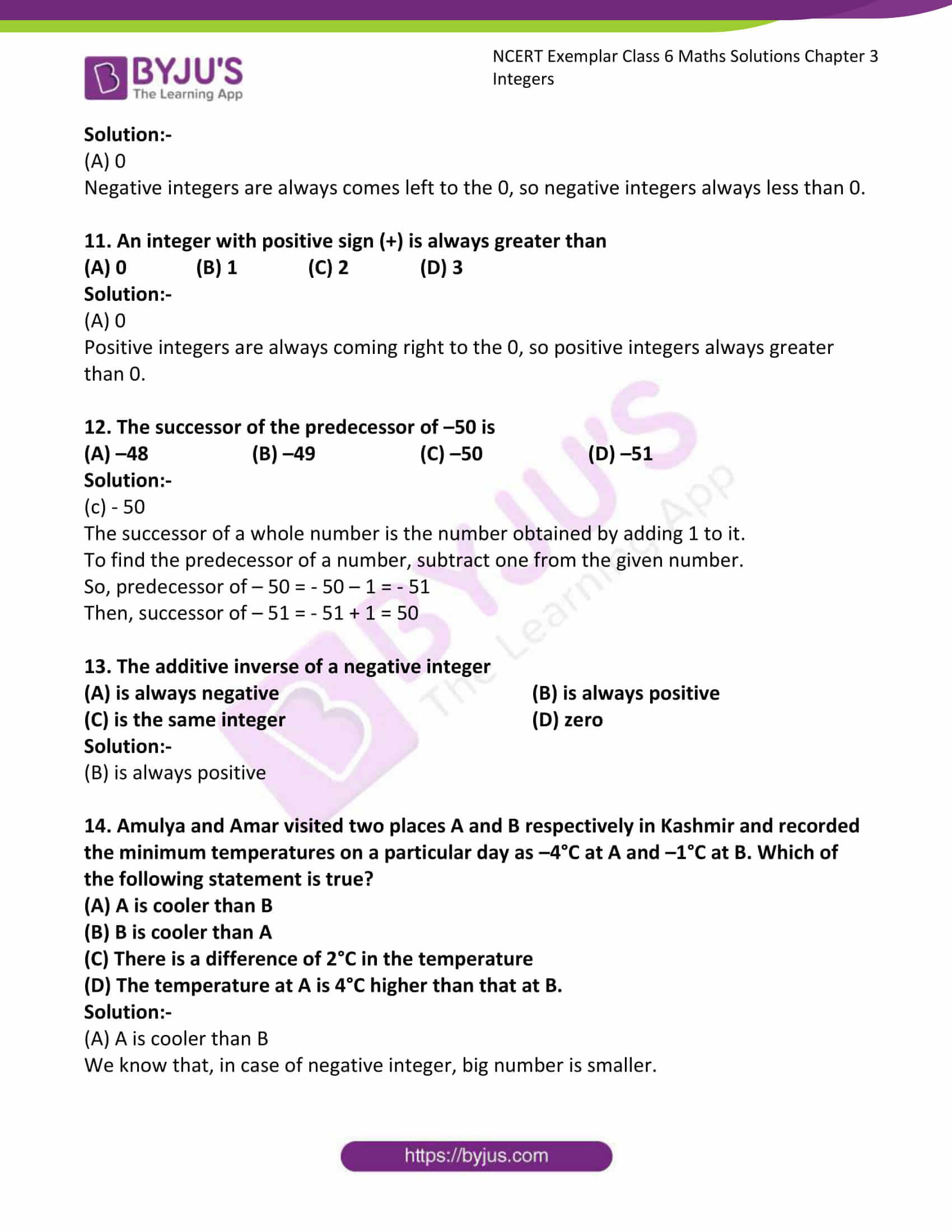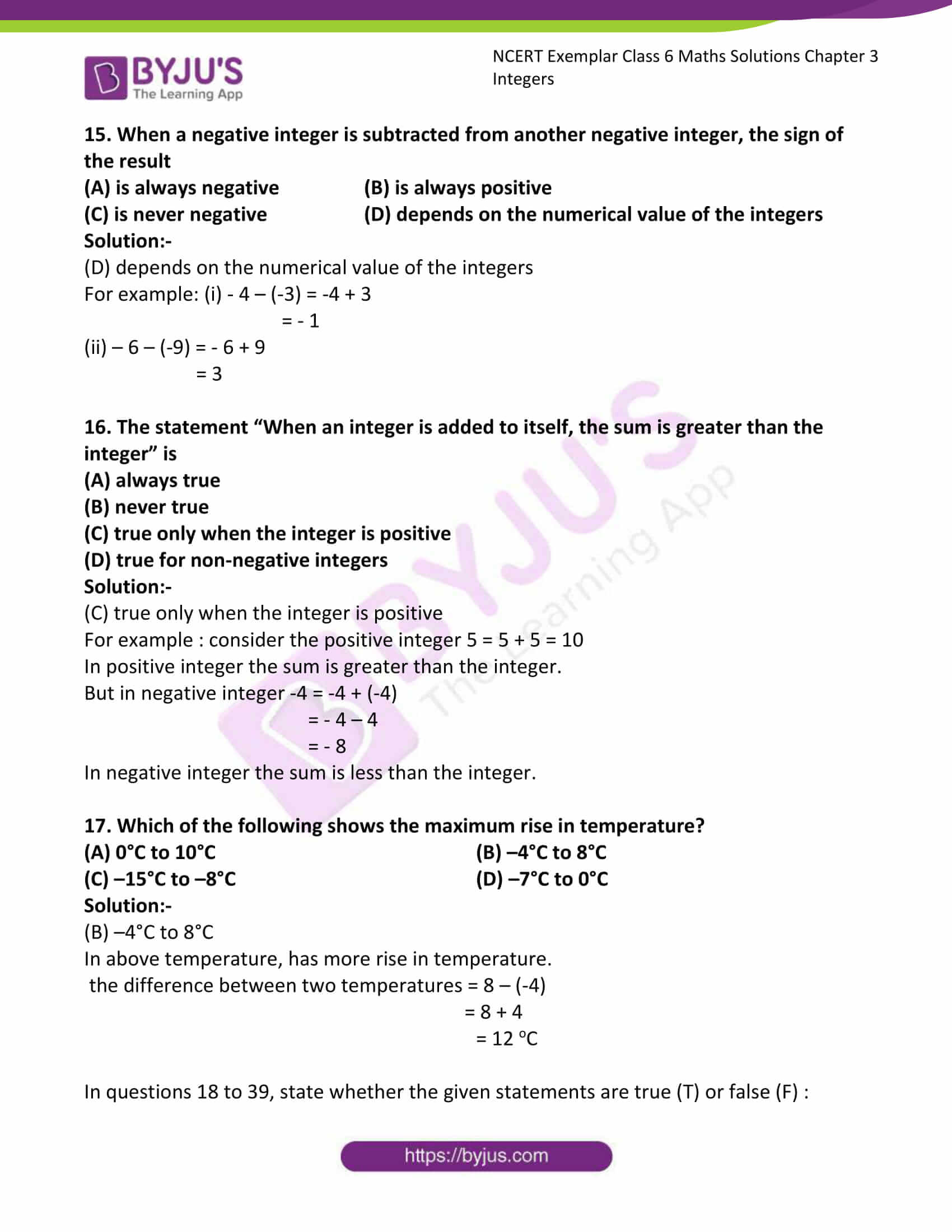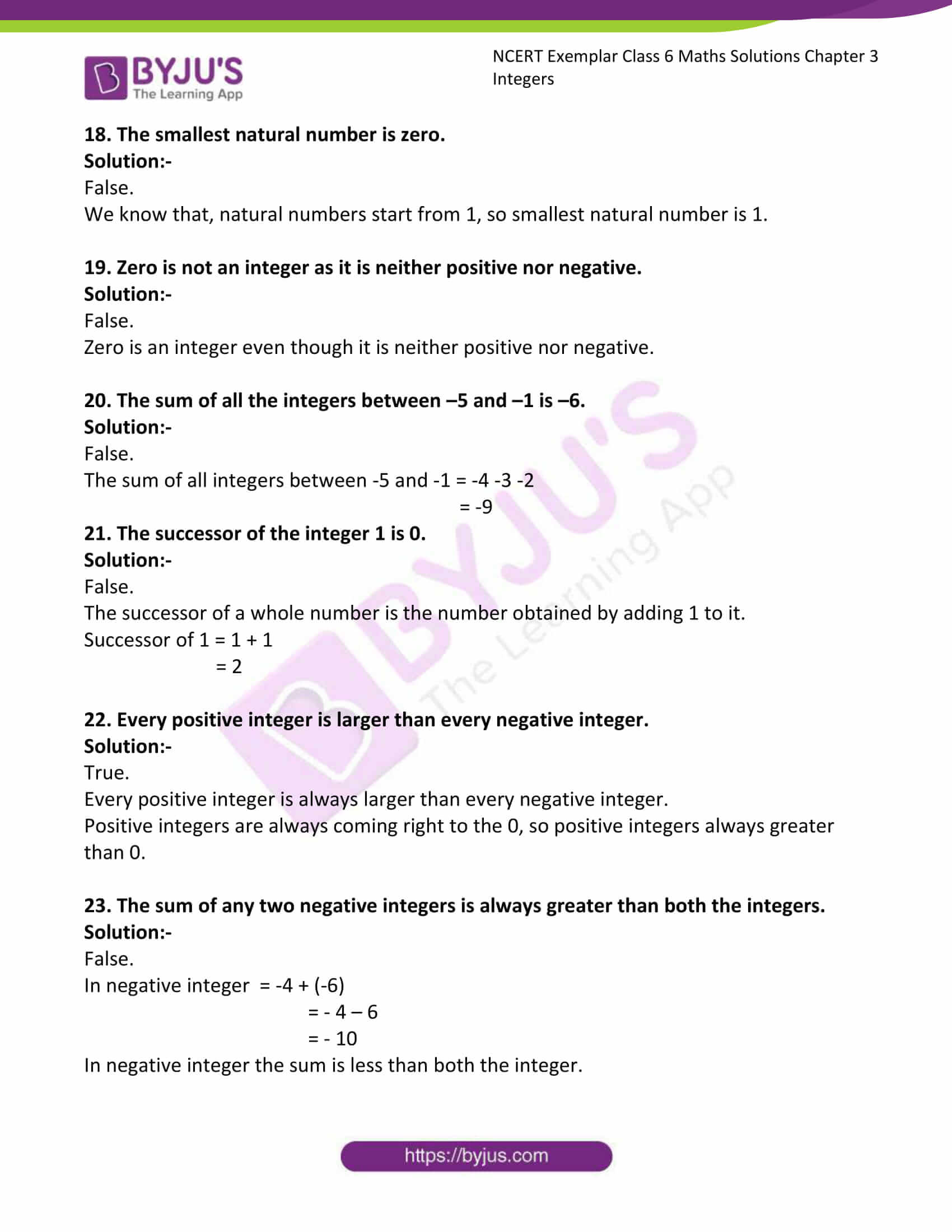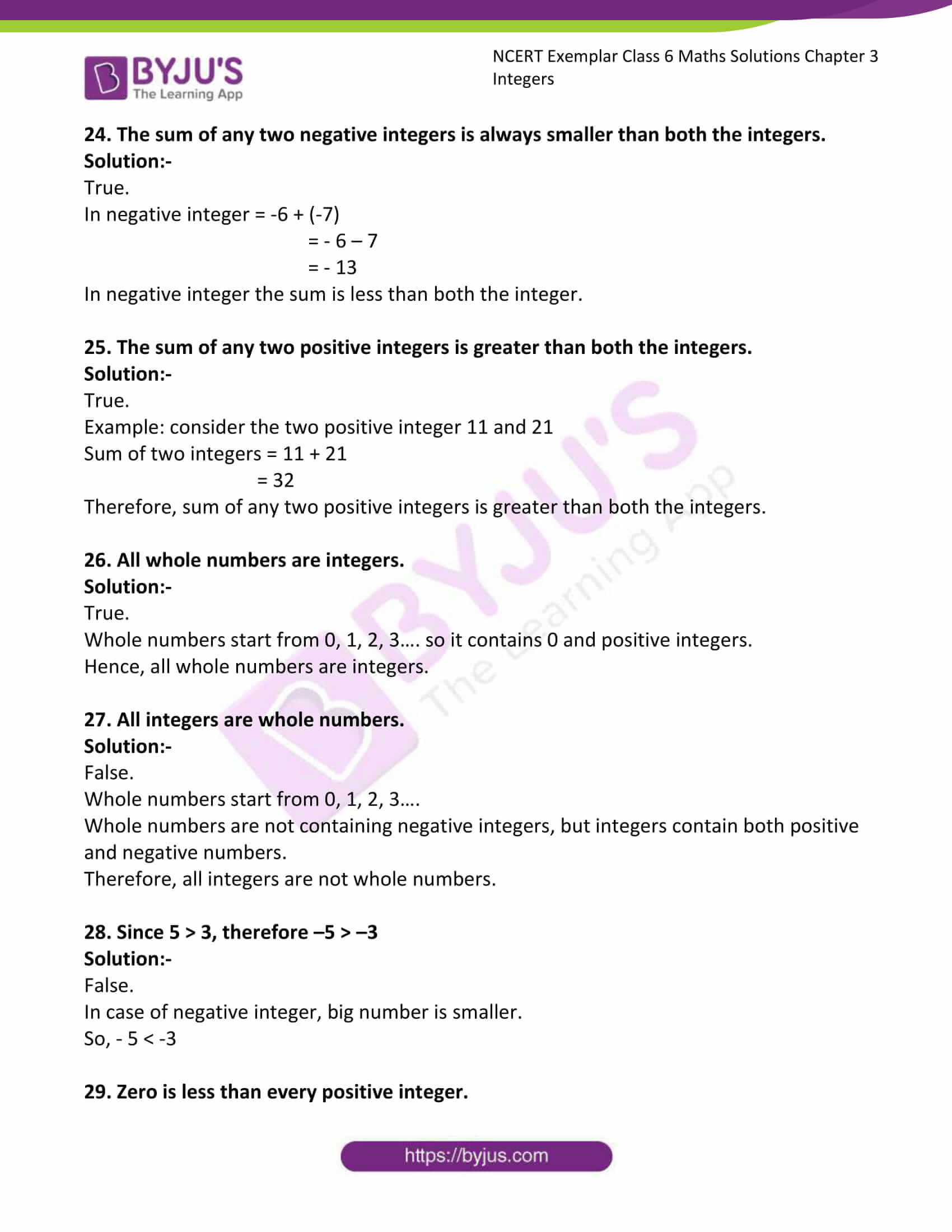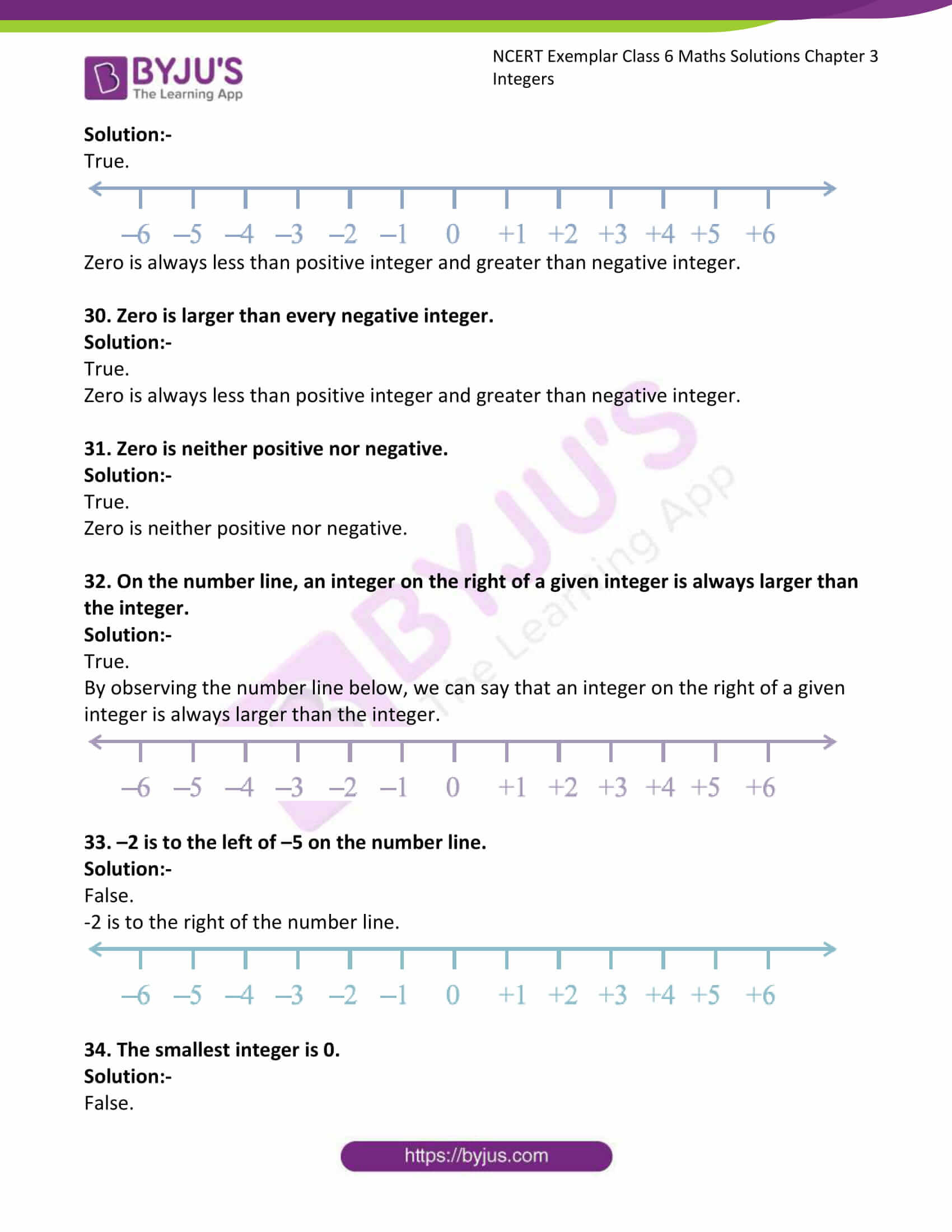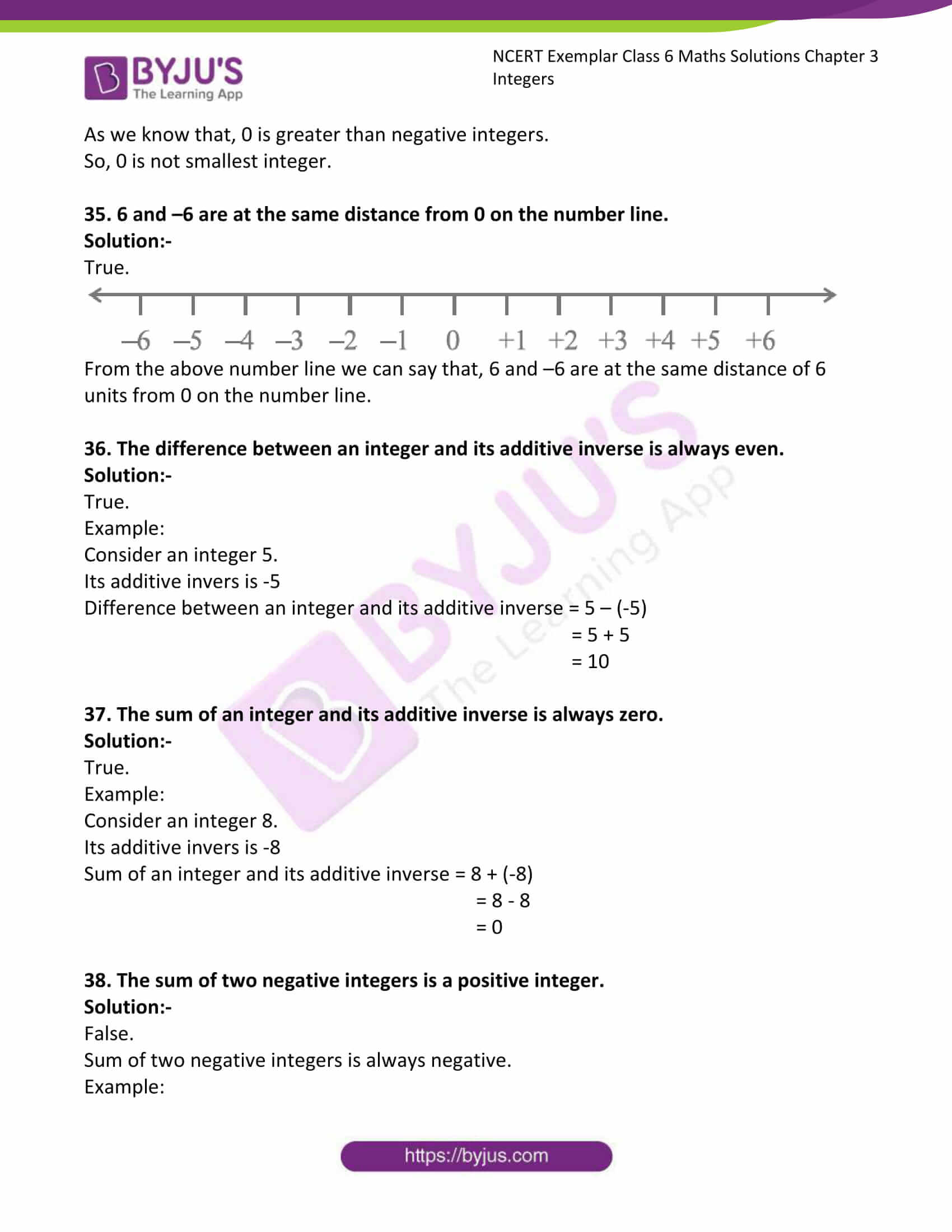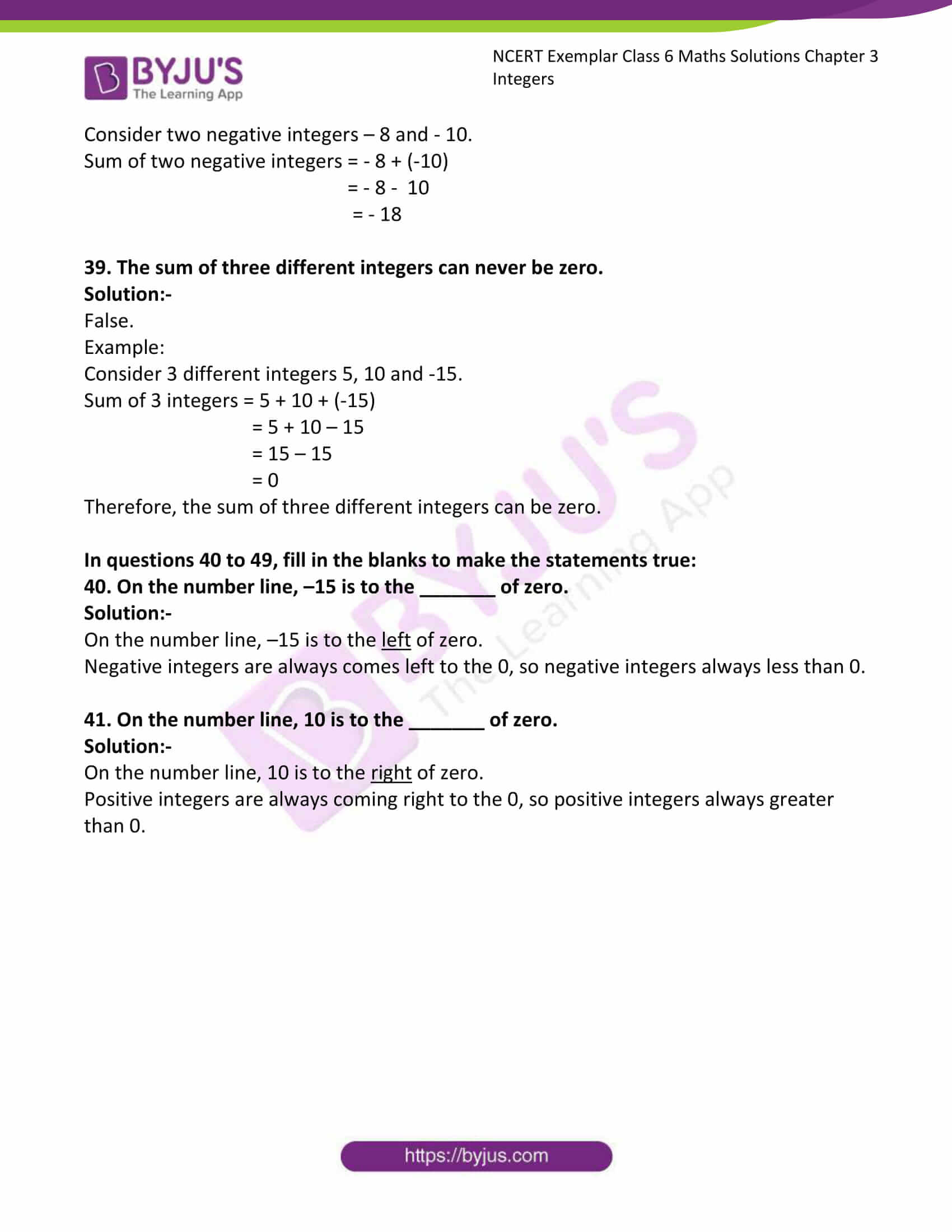### Access Answers to NCERT Exemplar Solutions for Class 6 Maths Chapter 3 Integers

Exercise Page: 45

In questions 1 to 17, only one of the four options is correct. Write the correct one.

1. Every integer less than 0 has the sign

(A) + (B) – (C) × (D) ÷

Solution:-

(B) –

The numbers –1, –2, –3, –4, ……. are referred to as negative integers.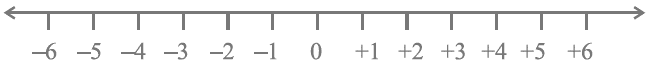All negative integers are less than zero.

2. The integer ‘5 units to the right of 0 on the number line’ is

(A) +5 (B) –5 (C) +4 (D) – 4

Solution:-

(A) +53. The predecessor of the integer –1 is

(A) 0 (B) 2 (C) –2 (D) 1

Solution:-

(C) –2

The number which comes immediately before a particular number is called its predecessor.

To find the predecessor of a number, subtract one from the given number.

So, predecessor of -1 = -1 -1 = -2

4. Number of integers lying between –1 and 1 is

(A) 1 (B) 2 (C) 3 (D) 0

Solution:-

(A) 1

Integer that comes between -1 and 1 is 0.

5. Number of whole numbers lying between –5 and 5 is

(A) 10 (B) 3 (C) 4 (D) 5

Solution:-

(D) 5

We know that, whole numbers are starts from 0.

Then, number of whole numbers between -5 and 5 are 0, 1, 2, 3, and 4.

6. The greatest integer lying between –10 and –15 is

(A) –10 (B) –11 (C) –15 (D) –14

Solution:-

(B) -11

In case of negative integer, small number is greater.

7. The least integer lying between –10 and –15 is

(A) –10 (B) –11 (C) –15 (D) –14

Solution:-

(D) –14

In case of negative integer, big number is smaller.

8. On the number line, the integer 5 is located

(A) to the left of 0 (B) to the right of 0

(C) to the left of 1 (D) to the left of –2

Solution:-

(B) to the right of 09. In which of the following pairs of integers, the first integer is not on the left of the other integer on the number line?

(A) (–1, 10) (B) (–3, –5) (C) (–5, –3) (D) (–6, 0)

Solution:-

(B) (–3, –5)10. The integer with negative sign (–) is always less than

(A) 0 (B) –3 (C) –1 (D) –2

Solution:-

(A) 0

Negative integers are always comes left to the 0, so negative integers always less than 0.

11. An integer with positive sign (+) is always greater than

(A) 0 (B) 1 (C) 2 (D) 3

Solution:-

(A) 0

Positive integers are always coming right to the 0, so positive integers always greater than 0.

12. The successor of the predecessor of –50 is

(A) –48 (B) –49 (C) –50 (D) –51

Solution:-

(c) – 50

The successor of a whole number is the number obtained by adding 1 to it.

To find the predecessor of a number, subtract one from the given number.

So, predecessor of – 50 = – 50 – 1 = – 51

Then, successor of – 51 = – 51 + 1 = 50

13. The additive inverse of a negative integer

(A) is always negative (B) is always positive

(C) is the same integer (D) zero

Solution:-

(B) is always positive

14. Amulya and Amar visited two places A and B respectively in Kashmir and recorded the minimum temperatures on a particular day as –4°C at A and –1°C at B. Which of the following statement is true?

(A) A is cooler than B

(B) B is cooler than A

(C) There is a difference of 2°C in the temperature

(D) The temperature at A is 4°C higher than that at B.

Solution:-

(A) A is cooler than B

We know that, in case of negative integer, big number is smaller.

15. When a negative integer is subtracted from another negative integer, the sign of the result

(A) is always negative (B) is always positive

(C) is never negative (D) depends on the numerical value of the integers

Solution:-

(D) depends on the numerical value of the integers

For example: (i) – 4 – (-3) = -4 + 3

= – 1

(ii) – 6 – (-9) = – 6 + 9

= 3

16. The statement “When an integer is added to itself, the sum is greater than the integer” is

(A) always true

(B) never true

(C) true only when the integer is positive

(D) true for non-negative integers

Solution:-

(C) true only when the integer is positive

For example : consider the positive integer 5 = 5 + 5 = 10

In positive integer the sum is greater than the integer.

But in negative integer -4 = -4 + (-4)

= – 4 – 4

= – 8

In negative integer the sum is less than the integer.

17. Which of the following shows the maximum rise in temperature?
(A) 0°C to 10°C (B) –4°C to 8°C

(C) –15°C to –8°C (D) –7°C to 0°C

Solution:-

(B) –4°C to 8°C

In above temperature, has more rise in temperature.

the difference between two temperatures = 8 – (-4)

= 8 + 4

= 12 oC

In questions 18 to 39, state whether the given statements are true (T) or false (F) :

18. The smallest natural number is zero.

Solution:-

False.

We know that, natural numbers start from 1, so smallest natural number is 1.

19. Zero is not an integer as it is neither positive nor negative.

Solution:-

False.

Zero is an integer even though it is neither positive nor negative.

20. The sum of all the integers between –5 and –1 is –6.

Solution:-

False.

The sum of all integers between -5 and -1 = -4 -3 -2

= -9

21. The successor of the integer 1 is 0.

Solution:-

False.

The successor of a whole number is the number obtained by adding 1 to it.

Successor of 1 = 1 + 1

= 2

22. Every positive integer is larger than every negative integer.

Solution:-

True.

Every positive integer is always larger than every negative integer.

Positive integers are always coming right to the 0, so positive integers always greater than 0.

23. The sum of any two negative integers is always greater than both the integers.

Solution:-

False.

In negative integer = -4 + (-6)

= – 4 – 6

= – 10

In negative integer the sum is less than both the integer.

24. The sum of any two negative integers is always smaller than both the integers.

Solution:-

True.

In negative integer = -6 + (-7)

= – 6 – 7

= – 13

In negative integer the sum is less than both the integer.

25. The sum of any two positive integers is greater than both the integers.

Solution:-

True.

Example: consider the two positive integer 11 and 21

Sum of two integers = 11 + 21

= 32

Therefore, sum of any two positive integers is greater than both the integers.

26. All whole numbers are integers.

Solution:-

True.

Whole numbers start from 0, 1, 2, 3…. so it contains 0 and positive integers.

Hence, all whole numbers are integers.

27. All integers are whole numbers.

Solution:-

False.

Whole numbers start from 0, 1, 2, 3….

Whole numbers are not containing negative integers, but integers contain both positive and negative numbers.

Therefore, all integers are not whole numbers.

28. Since 5 > 3, therefore –5 > –3

Solution:-

False.

In case of negative integer, big number is smaller.

So, – 5 < -3

29. Zero is less than every positive integer.

Solution:-

True.Zero is always less than positive integer and greater than negative integer.

30. Zero is larger than every negative integer.

Solution:-

True.

Zero is always less than positive integer and greater than negative integer.

31. Zero is neither positive nor negative.

Solution:-

True.

Zero is neither positive nor negative.

32. On the number line, an integer on the right of a given integer is always larger than the integer.

Solution:-

True.

By observing the number line below, we can say that an integer on the right of a given integer is always larger than the integer.33. –2 is to the left of –5 on the number line.

Solution:-

False.

-2 is to the right of the number line.34. The smallest integer is 0.

Solution:-

False.

As we know that, 0 is greater than negative integers.

So, 0 is not smallest integer.

35. 6 and –6 are at the same distance from 0 on the number line.

Solution:-

True.From the above number line we can say that, 6 and –6 are at the same distance of 6 units from 0 on the number line.

36. The difference between an integer and its additive inverse is always even.

Solution:-

True.

Example:

Consider an integer 5.

Difference between an integer and its additive inverse = 5 – (-5)

= 5 + 5

= 10

37. The sum of an integer and its additive inverse is always zero.

Solution:-

True.

Example:

Consider an integer 8.

Sum of an integer and its additive inverse = 8 + (-8)

= 8 – 8

= 0

38. The sum of two negative integers is a positive integer.

Solution:-

False.

Sum of two negative integers is always negative.

Example:

Consider two negative integers – 8 and – 10.

Sum of two negative integers = – 8 + (-10)

= – 8 – 10

= – 18

39. The sum of three different integers can never be zero.

Solution:-

False.

Example:

Consider 3 different integers 5, 10 and -15.

Sum of 3 integers = 5 + 10 + (-15)

= 5 + 10 – 15

= 15 – 15

= 0

Therefore, the sum of three different integers can be zero.

In questions 40 to 49, fill in the blanks to make the statements true:

40. On the number line, –15 is to the _______ of zero.

Solution:-

On the number line, –15 is to the left of zero.

Negative integers are always comes left to the 0, so negative integers always less than 0.

41. On the number line, 10 is to the _______ of zero.

Solution:-

On the number line, 10 is to the right of zero.

Positive integers are always coming right to the 0, so positive integers always greater than 0.